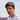# Douglas-Peucker algorithm

August 13, 2016

This post is a part of the series about shapes recognition. This post is also available as a part of a playground.

When drawing by hand, especially in a slow manner, we will get a lot of touch points and the resulting curve will contain lots of close points. Also we can not draw ideally, so resulting curve can contain artifacts like hooklet at the end of the curve, closed shapes can not be ideally closed. These artifacts are insignificant for recognizing a shape and only complicate calculations. To eliminate deviation from “ideal” curve there are different preprocessing algorithms that we can apply before proceeding to the next steps of shape recognition.

### Douglas-Peucker algorithm

When implementing smooth freehand drawing we were making our curve more complex by transforming line segments to Bezier curves. Now we will do opposite procedure - we will simplify our curve by removing insignificant points. Douglas-Peucker algorithm is one of the tools to solve that problem.

The purpose of the algorithm is, given a curve composed of line segments, to find a similar curve with fewer points. The algorithm defines ‘dissimilar’ based on the maximum distance between the original curve and the simplified curve (i.e., the Hausdorff distance between the curves). The simplified curve consists of a subset of the points that defined the original curve.1

The input of the algorithm is a curve represented by an ordered set of points (P1,…,Pn) and the threshold ℇ > 0. The output is the curve represented by the subset of the input set of points.

On the first step of the algorithm we search for the farthest point (Pz) from the line segment between the start and the end points (P1 and Pn). If that point is closer than the threshold (ℇ) all the points between P1 and Pn are discarded. Otherwise the Pz is included in the resulting set. Then we repeat the same step recursively with the right and the left parts of the curve (from P1 to Pz and from Pz to Pn). Then we merge the results of processing the left and the right parts. Algorithm repeats until all the points are handled.Simplifying a piecewise linear curve with the Douglas–Peucker algorithm.2

First let’s write a helper function that will find the farthest point.

For brevity I don’t include functions that are used to calculate the distance between the point and the line segment. They are pretty trivial geometric calculations and you can find them in the source files for this playground.

``````    typealias FarthestPoint = (index: Int, point: CGPoint!, distance: CGFloat)

func farthestPoint(points: [CGPoint]) -> FarthestPoint? {
var farthest: FarthestPoint?

//if there are less then two points in the set return nil
guard points.count >= 3 else { return farthest }

let (p1, pn) = (points.first!, points.last!)

//find the farthest point from the line segment between the start and the end points
for i in 1..<(points.count - 1) {
let distance = points[i].distance(to: (p1, pn))
if distance > (farthest?.distance ?? -CGFloat.max) {
farthest = (i, points[i], distance)
}
}
return farthest
}``````

With that function it is trivial to implement the algorithm.

``````     func douglasPeucker(points: [CGPoint], tolerance: CGFloat) -> [CGPoint] {
//if the farthest point can not be found include all points from the input set
guard let farthest = farthestPoint(points) else {
return points
}
//if the farthest point is closer than a threshold only include the start and the end points of the input set
guard farthest.distance > tolerance else {
return [points.first!, points.last!]
}

//Otherwise recursively apply the algorithm to the left and to the right parts of the set
let left = douglasPeucker(Array(points[0...farthest.index]), tolerance: tolerance)
let right = douglasPeucker(Array(points[farthest.index..<points.count]), tolerance: tolerance)

//Now merge left and right parts removing duplicated point
return Array([Array(left.dropLast()), right].flatten())
}``````

Let’s see how this algorithm works on a sample set of points.
First here is the input path built by connecting each sample point with line segments:

Here is the resulting path with threshold value of 5. You can play with it and see how higher values will discard more points.

Here you can see how each step of the algorithm performs. The farthest point on the each step is marked with a square. Points discarded on a previous step are drawn with a cross.The algorithm can be implemented without recursion but I will leave it for you as an exercise.

Hint: Usually recursion can be replaced by using stacks.Ilya Puchka
iOS developer @Babylon